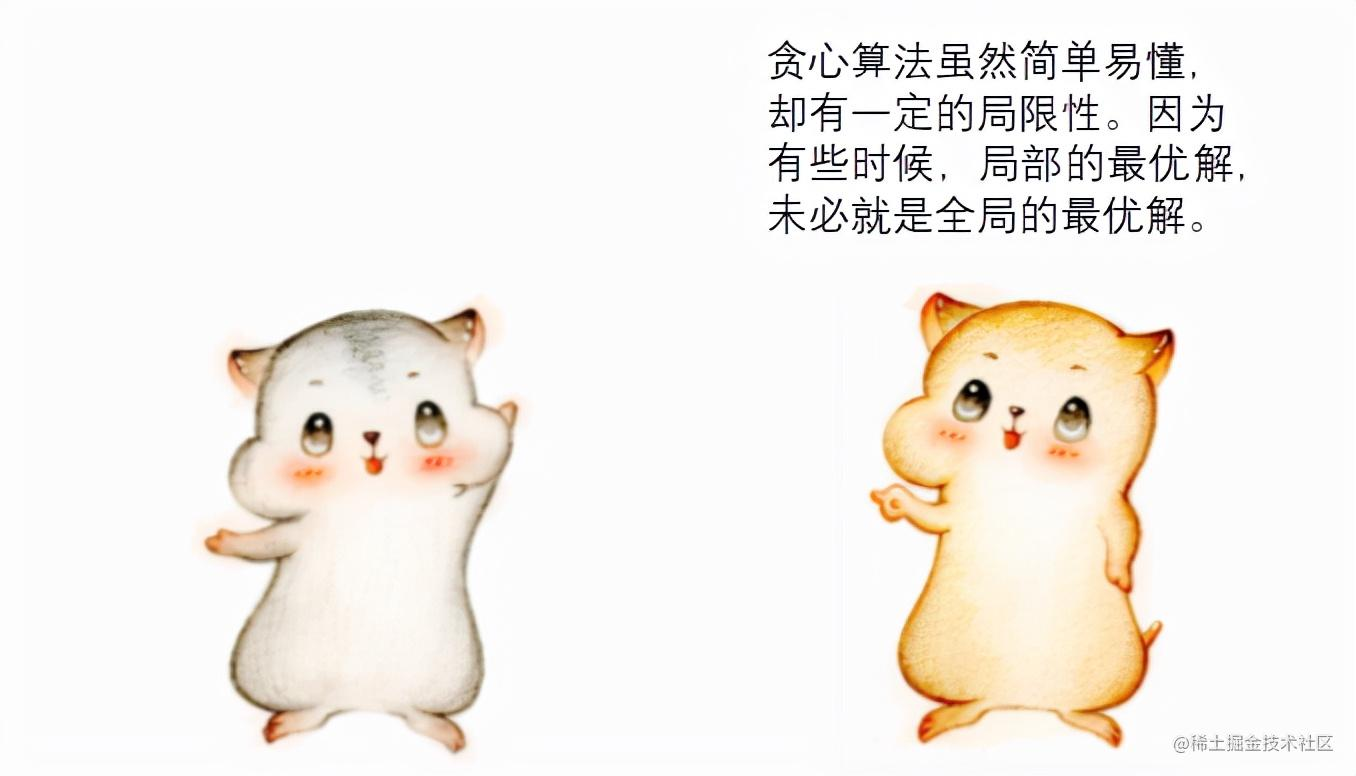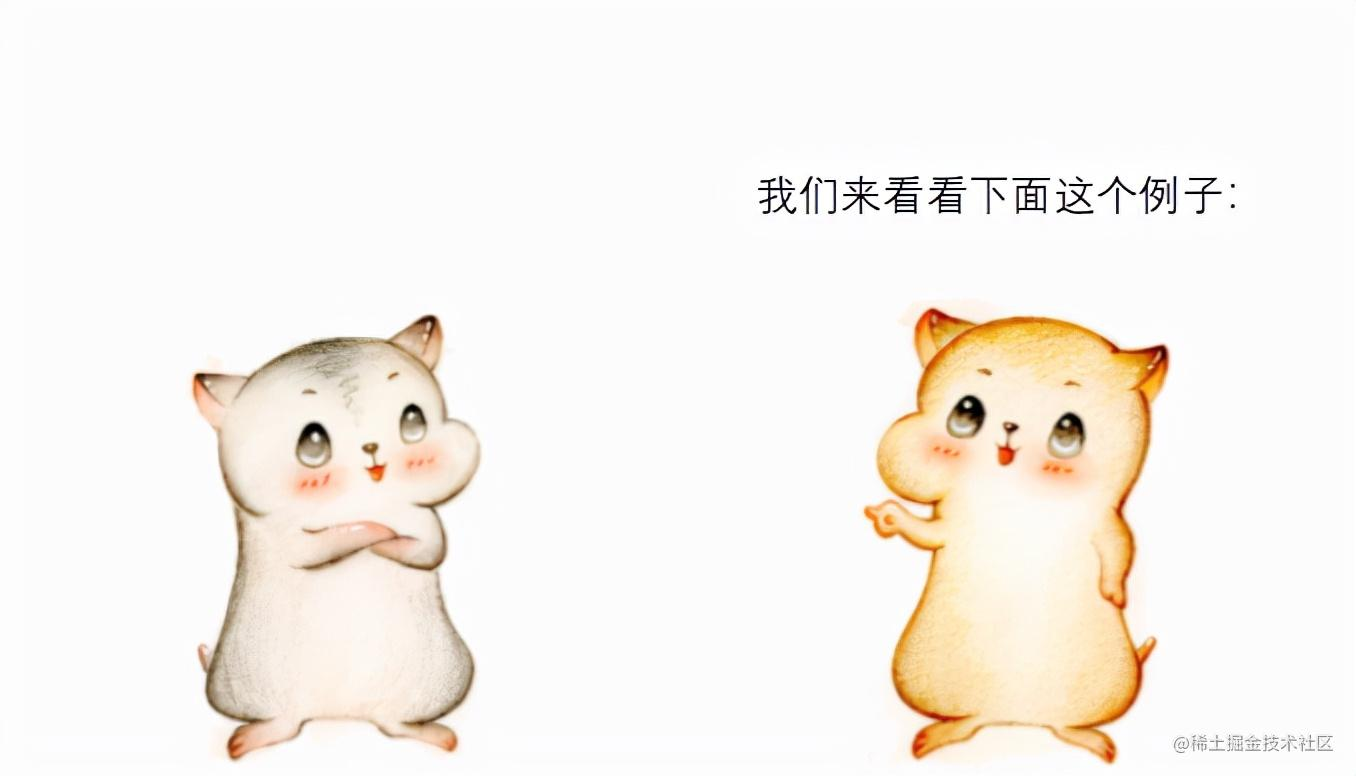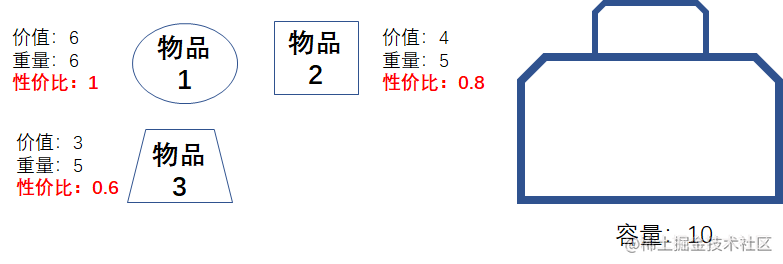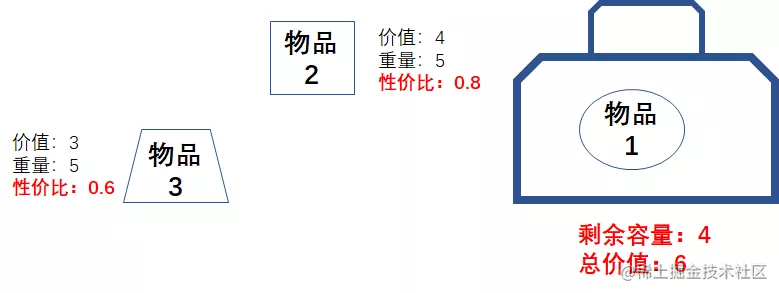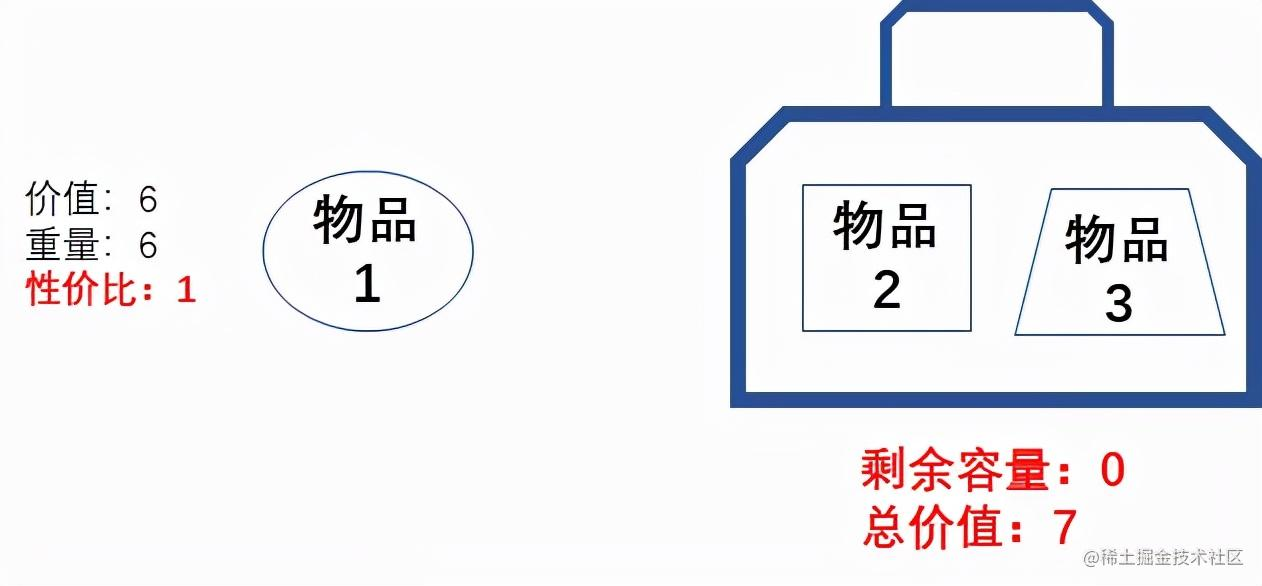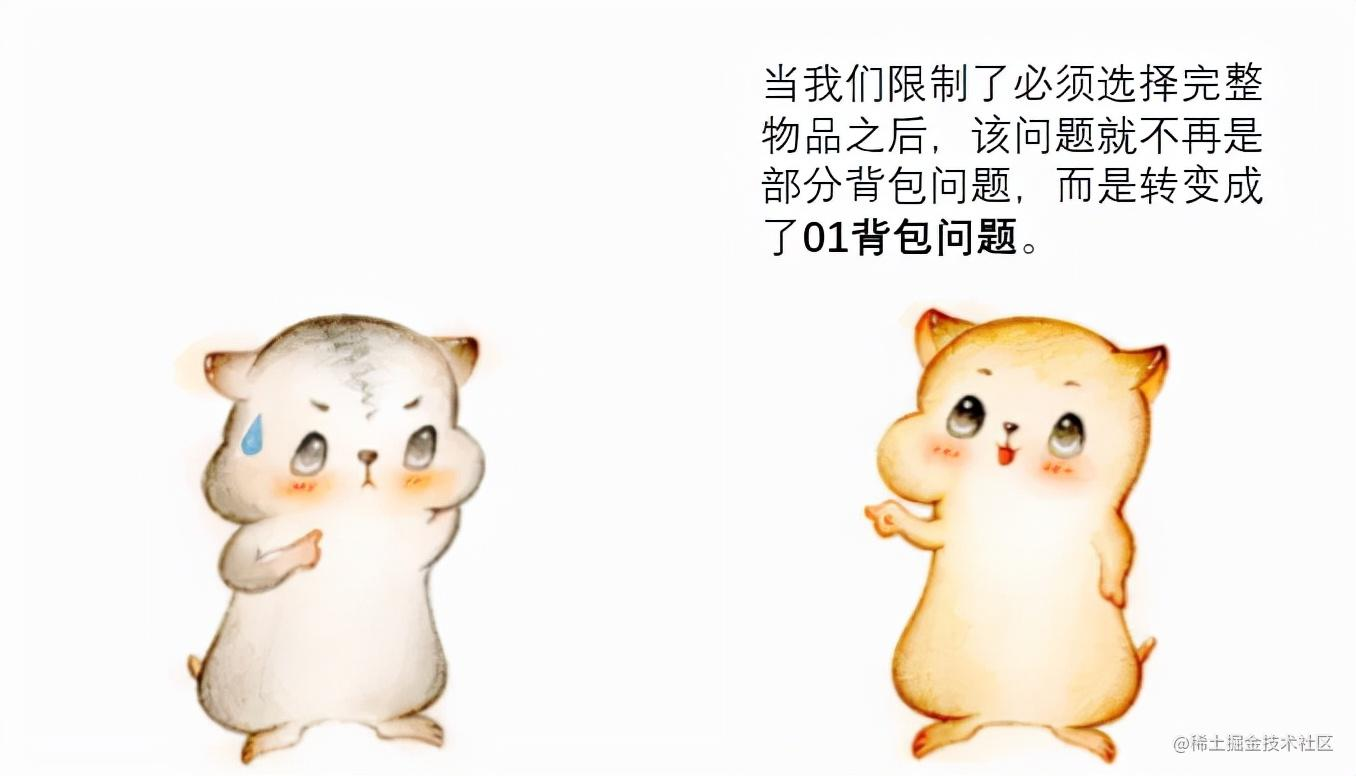漫画：什么是“贪心算法”？如何求解“部分背包问题”？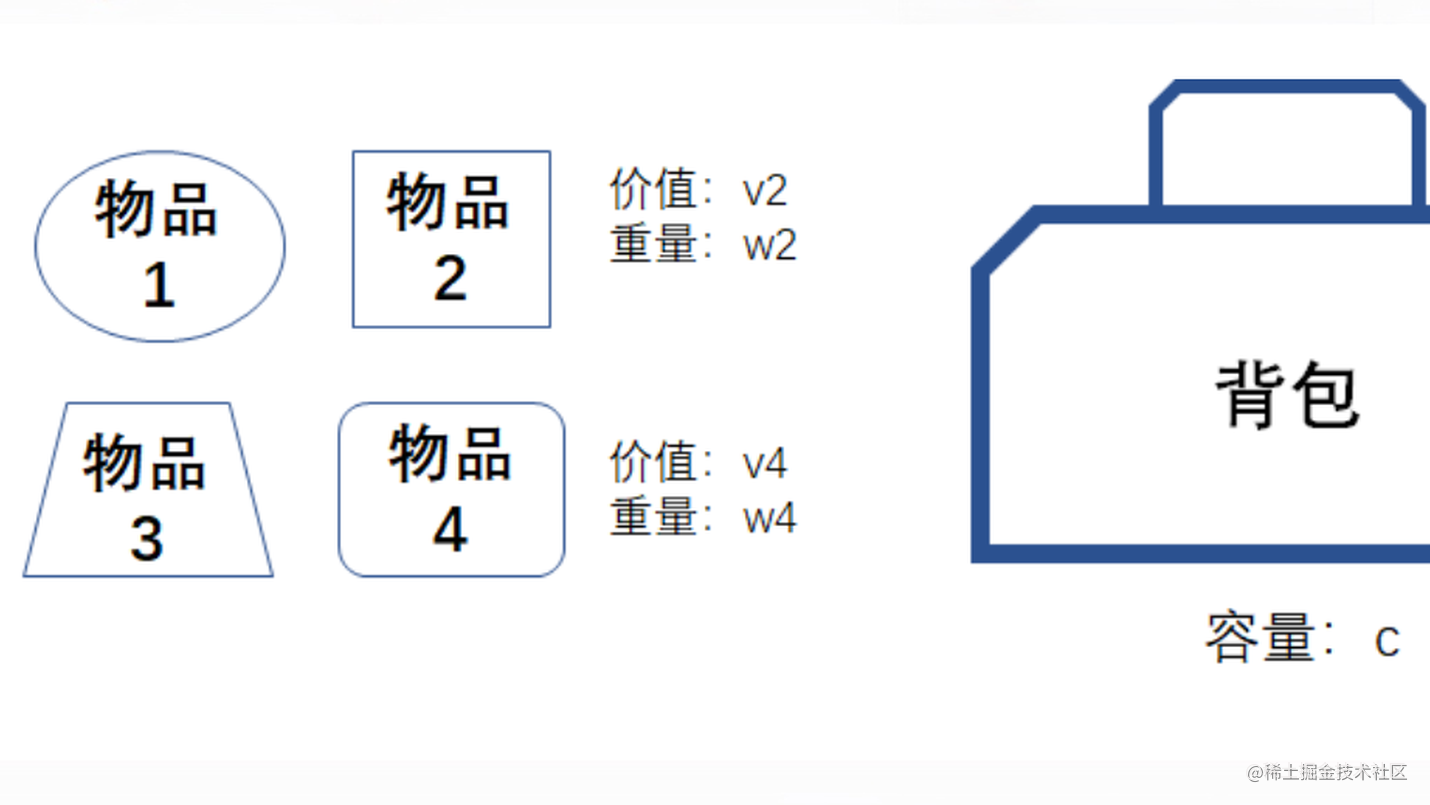————— 第二天 —————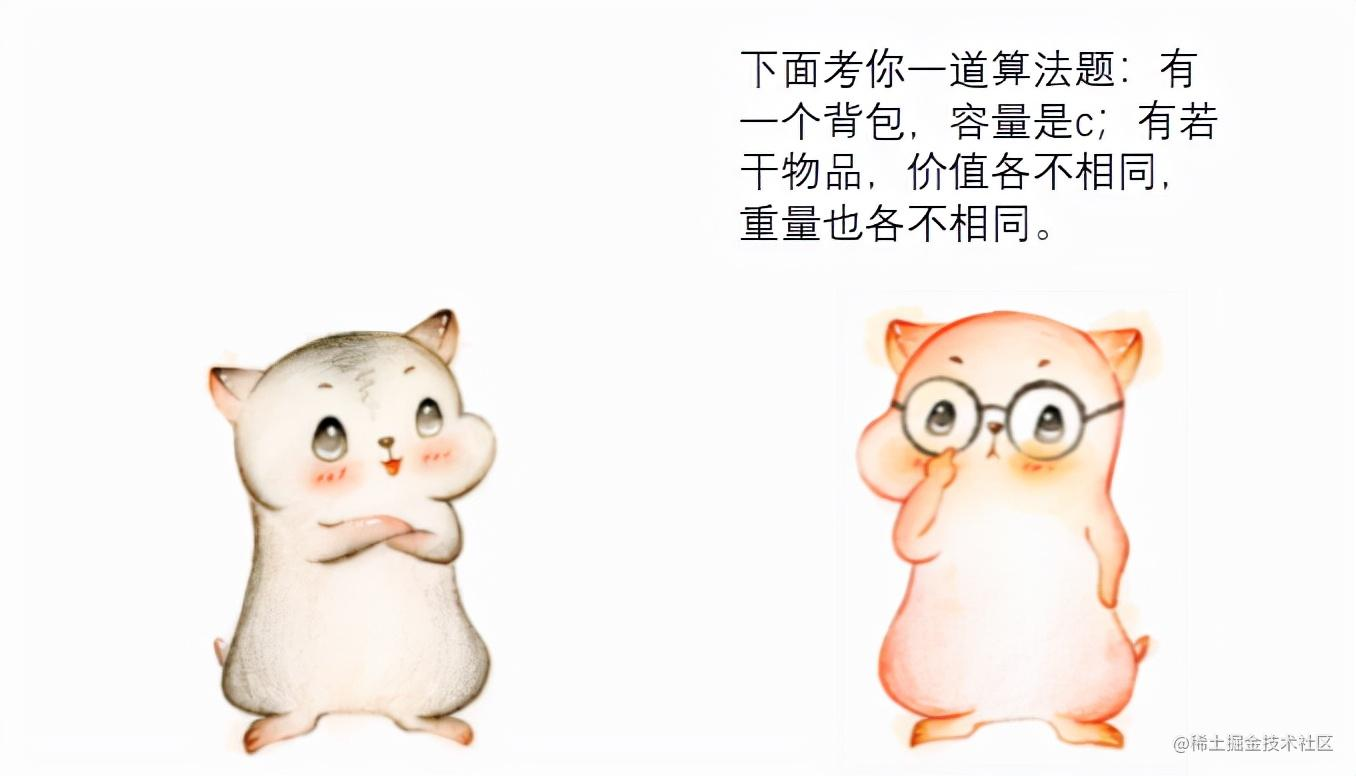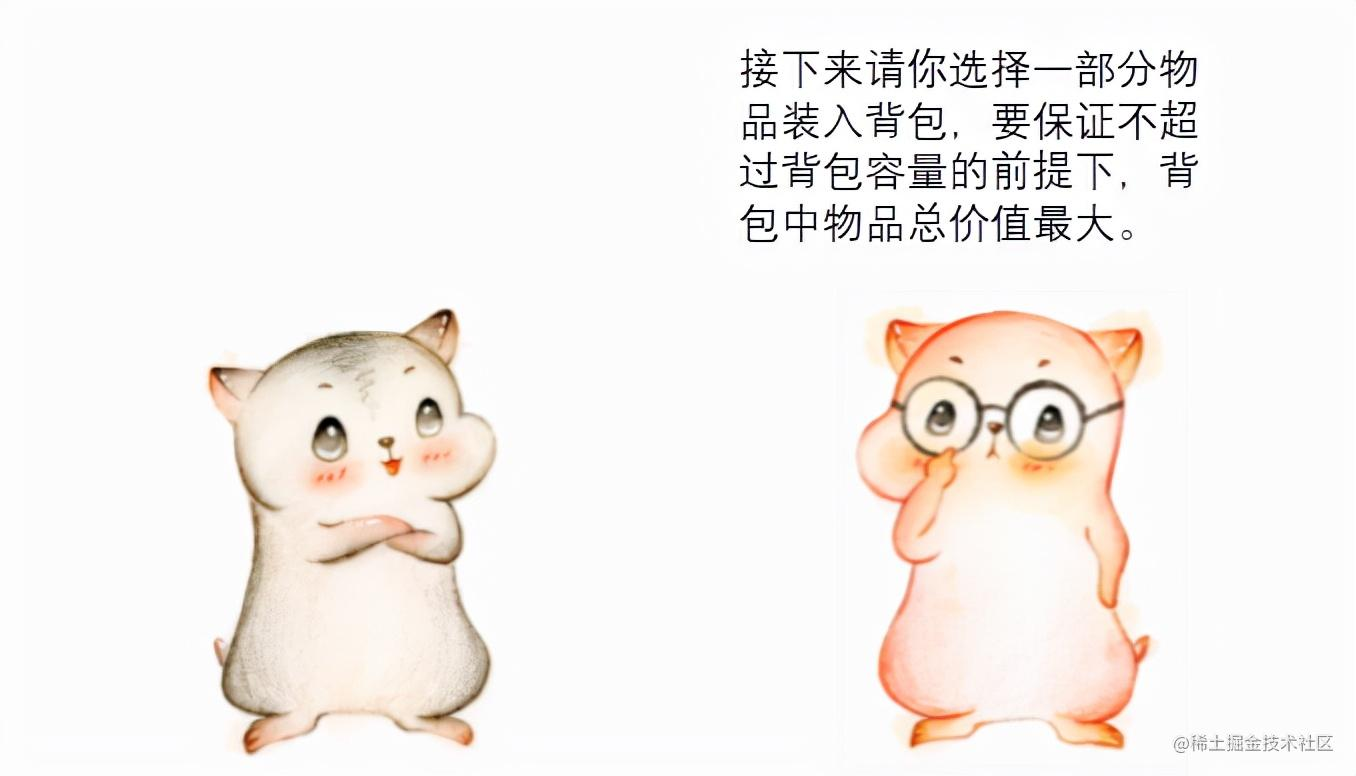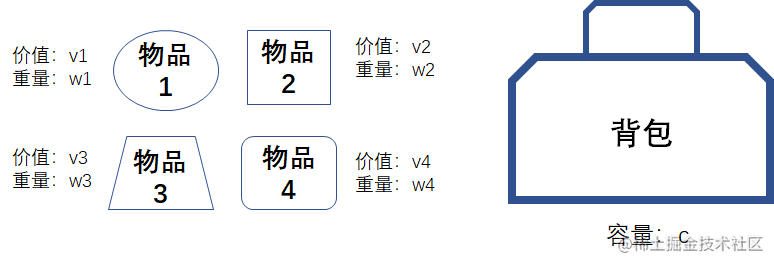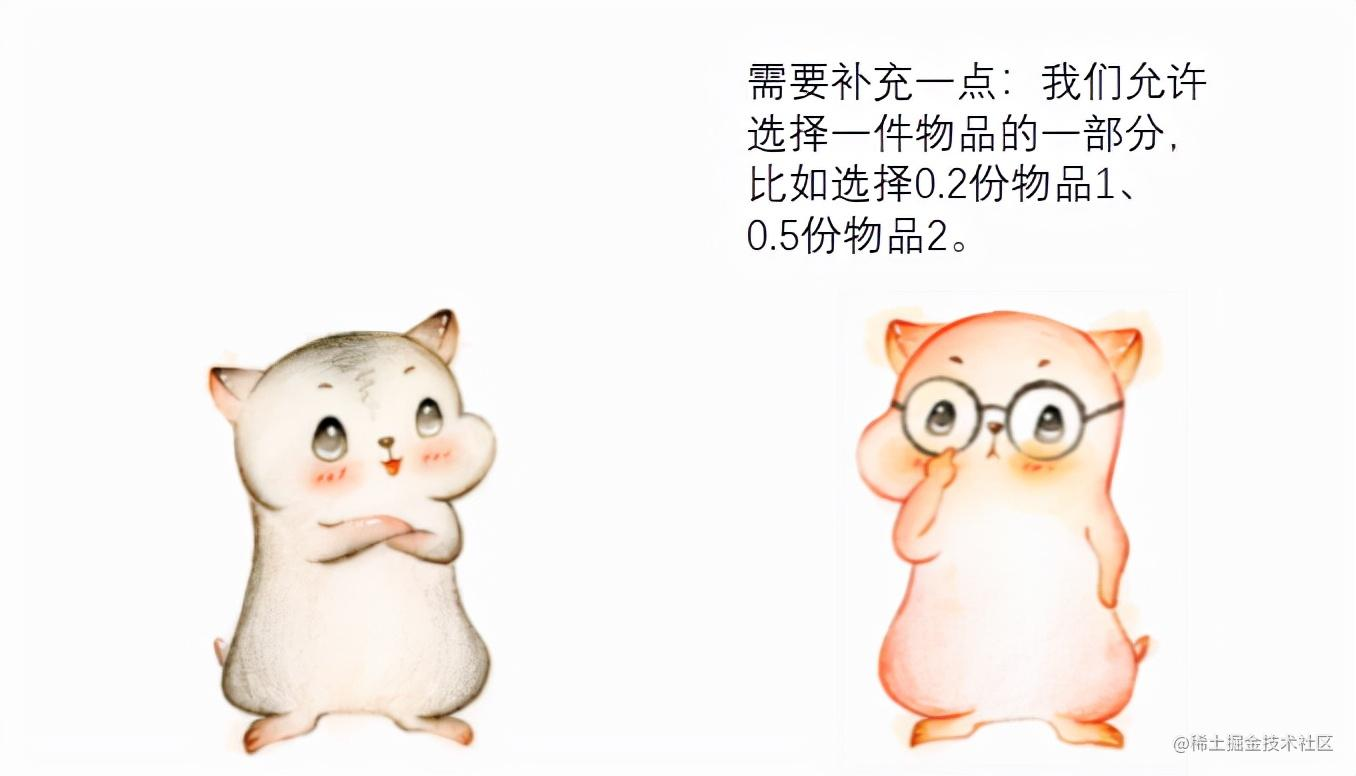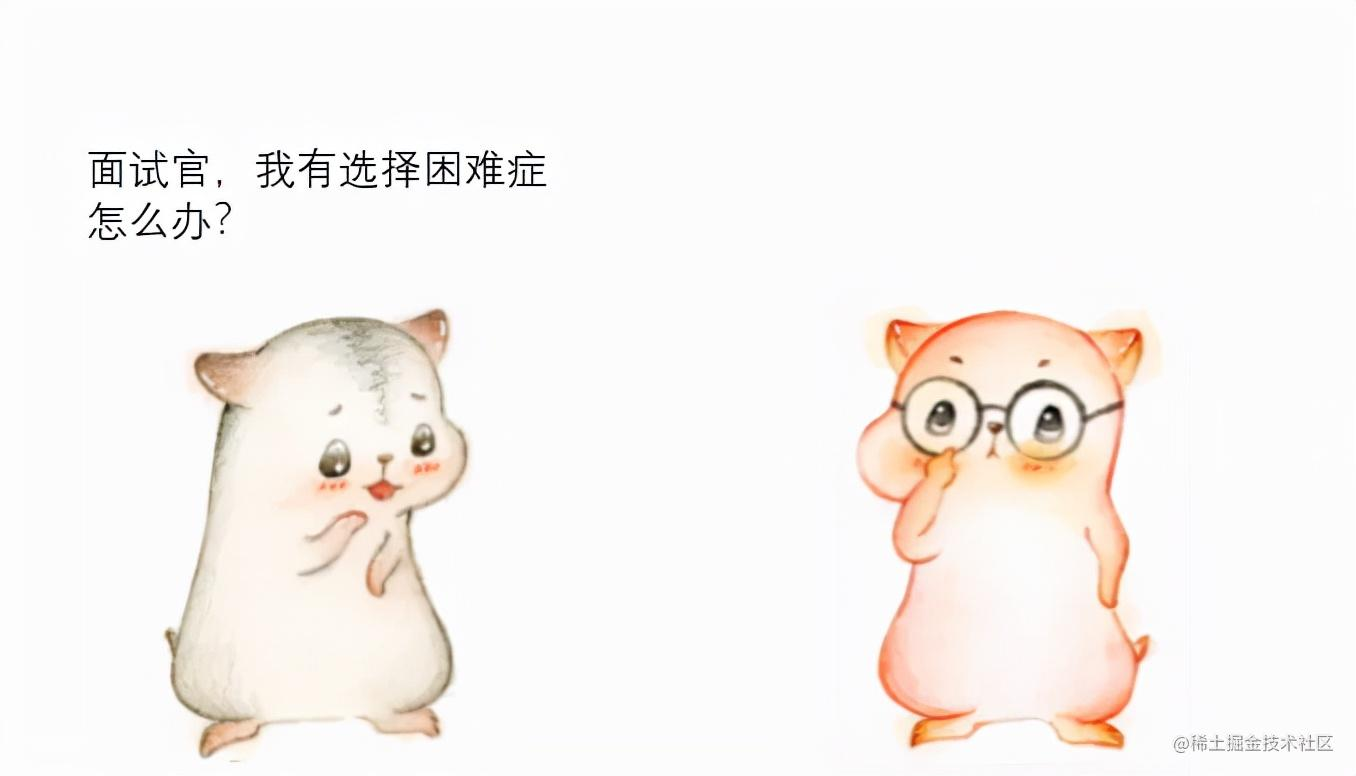————————————. . . . . . . .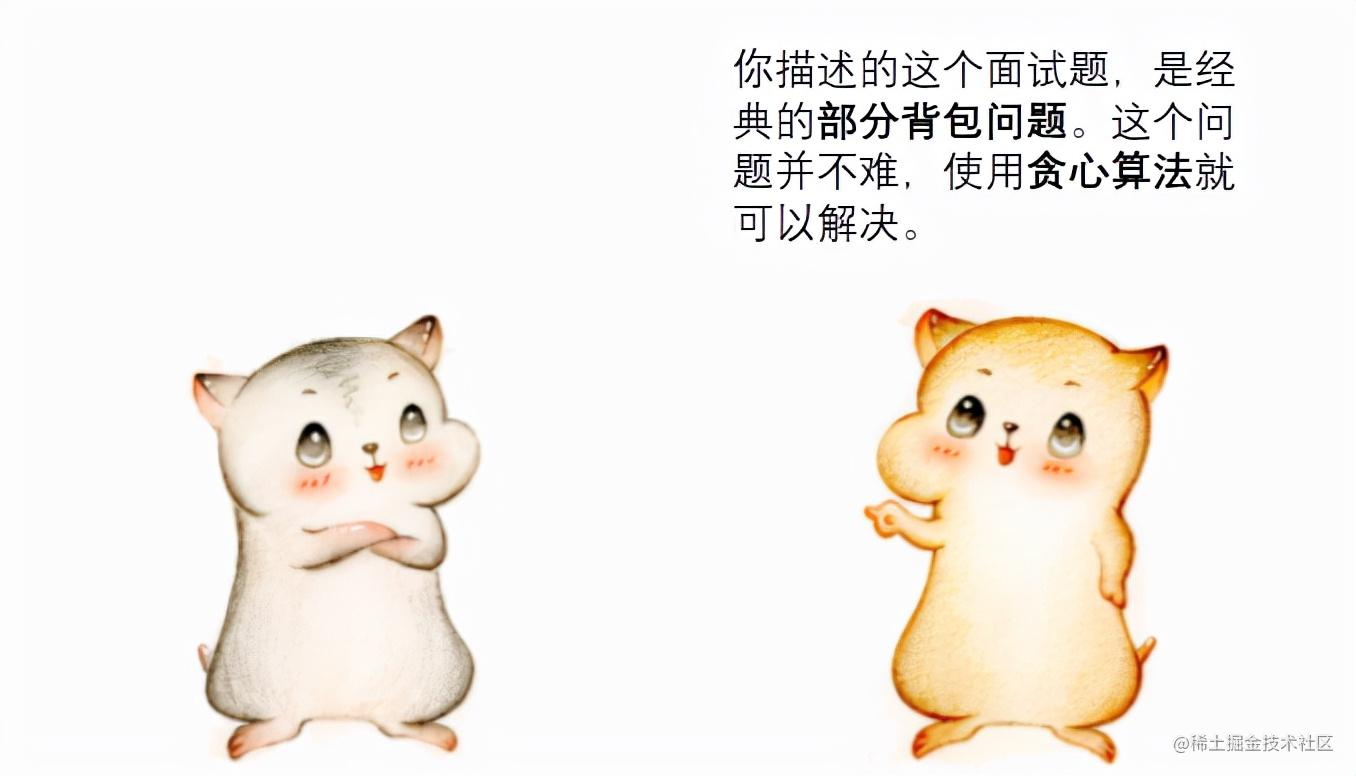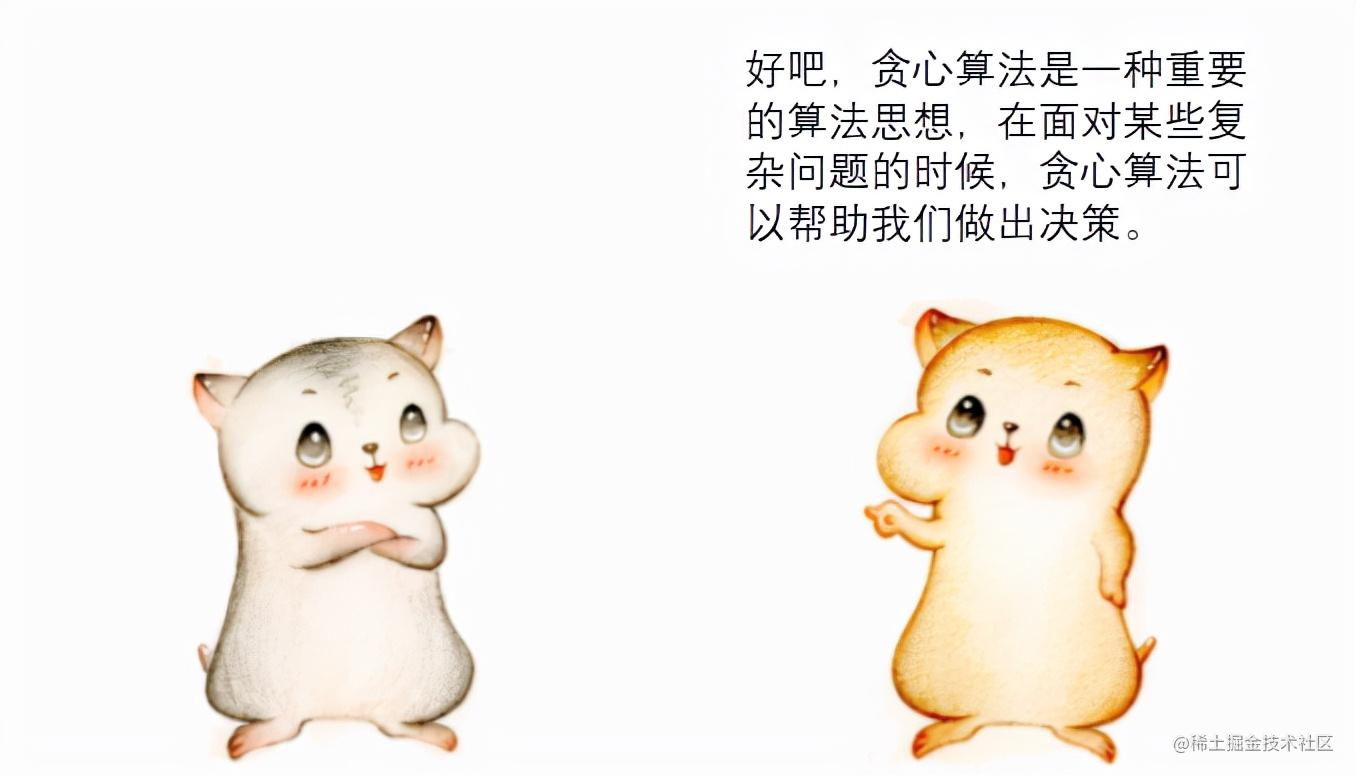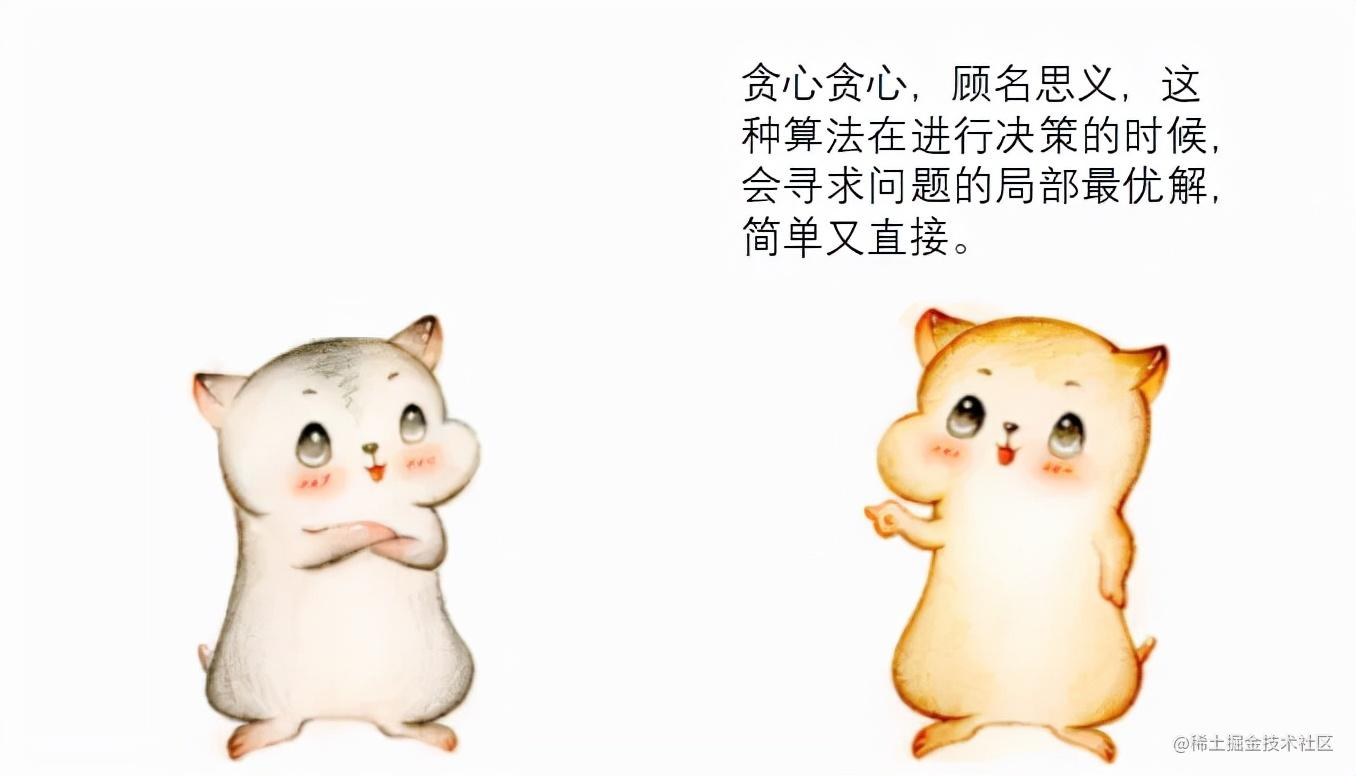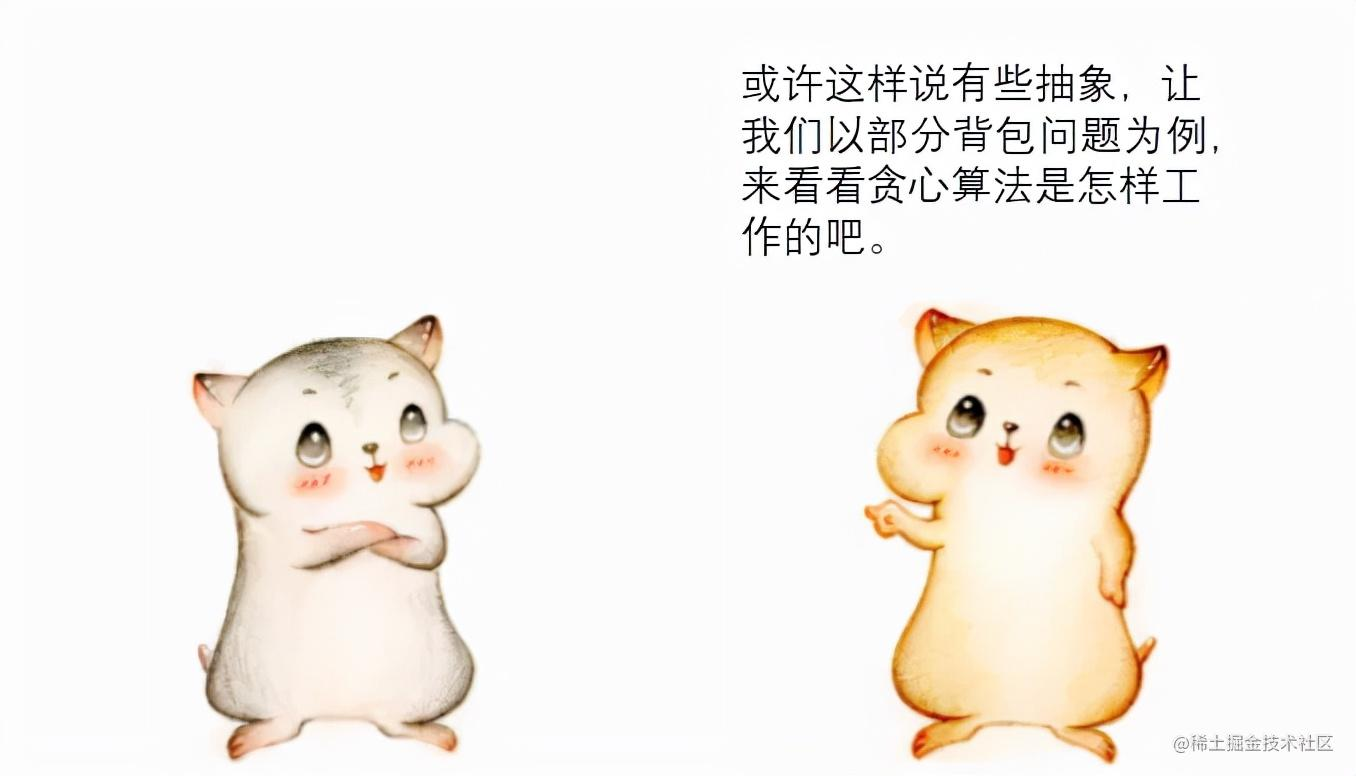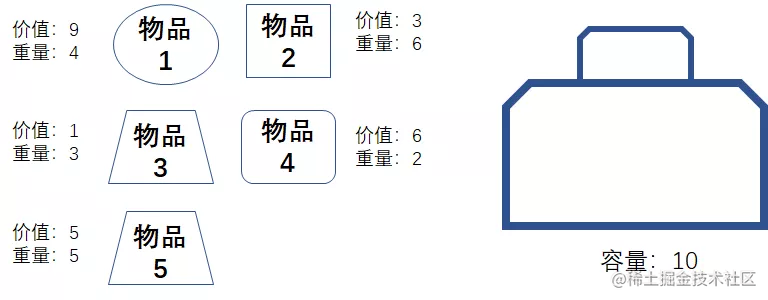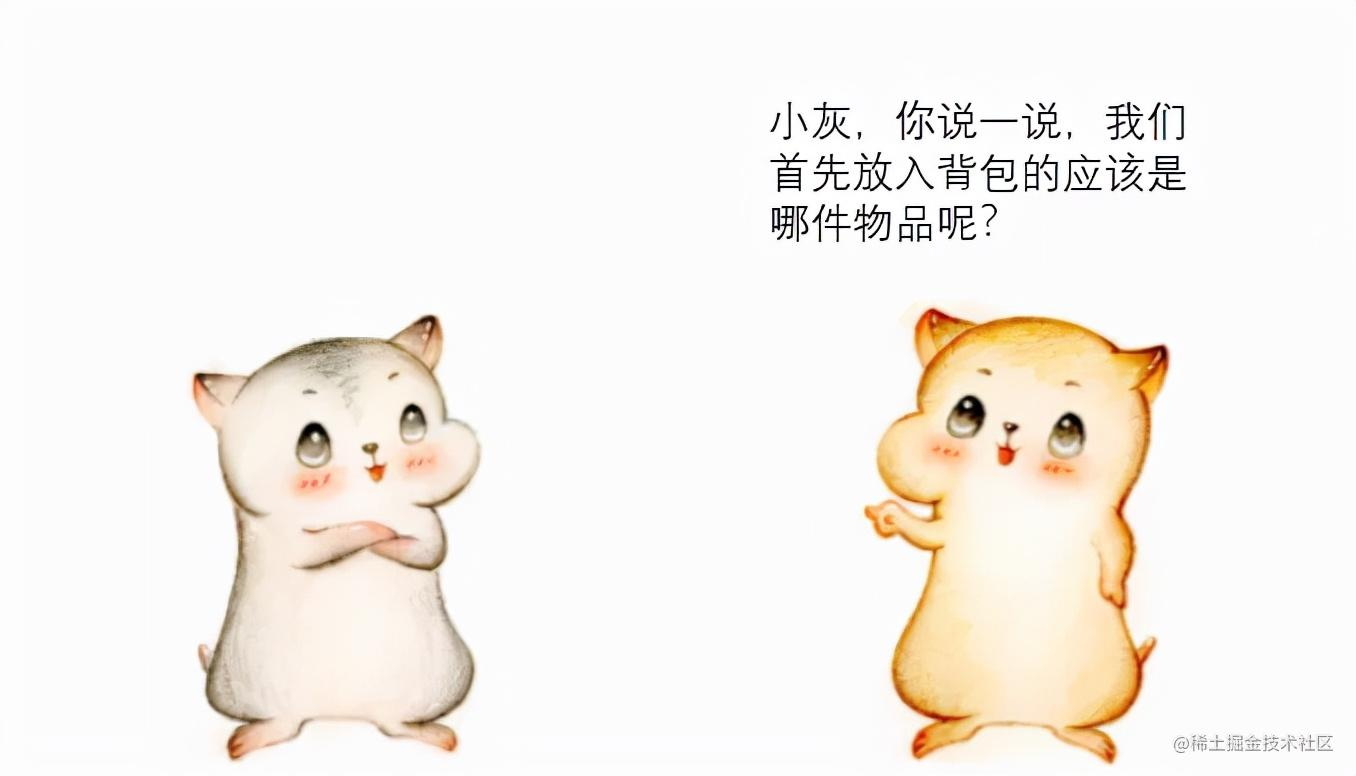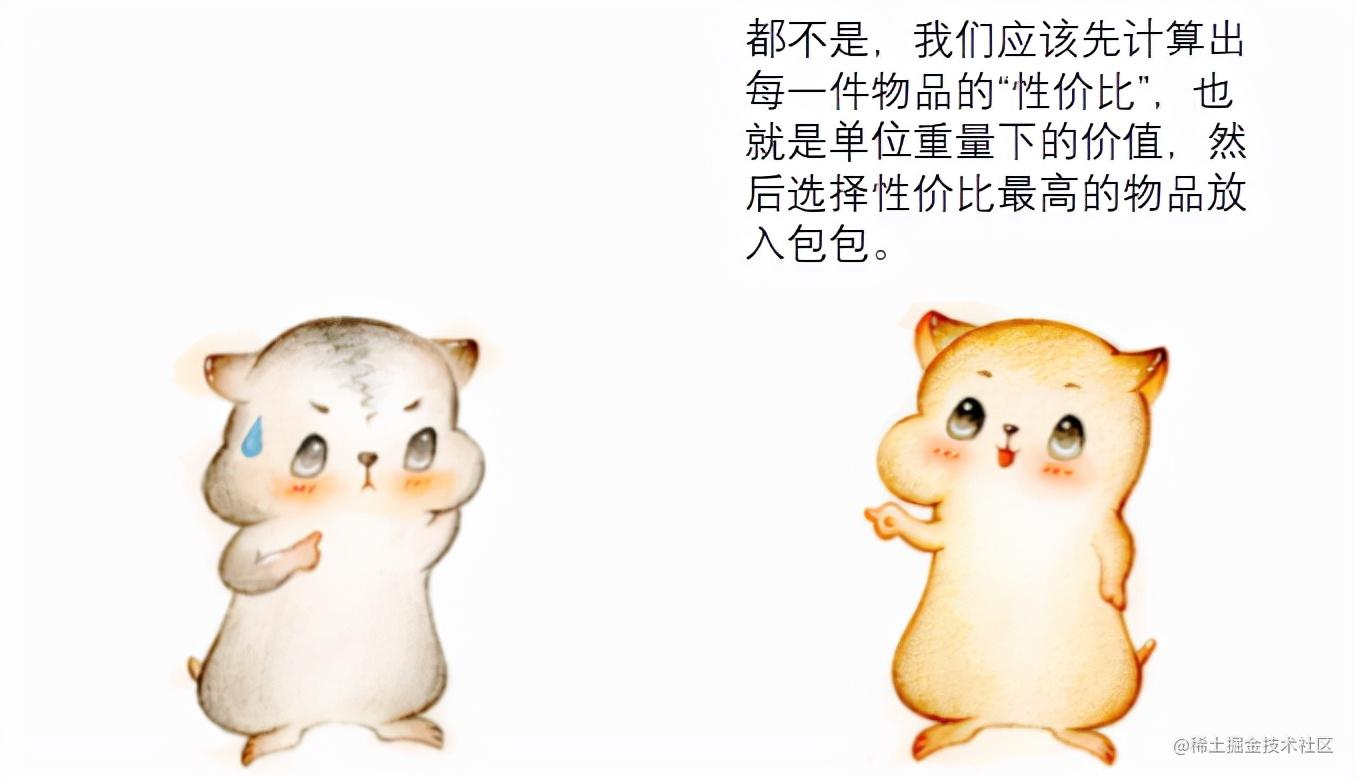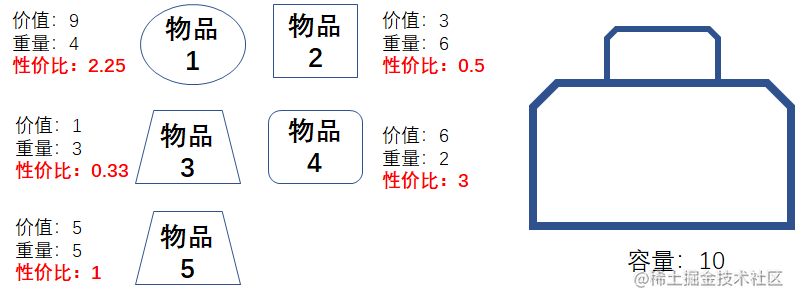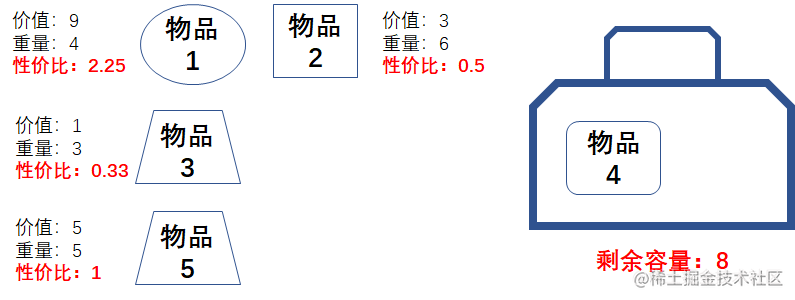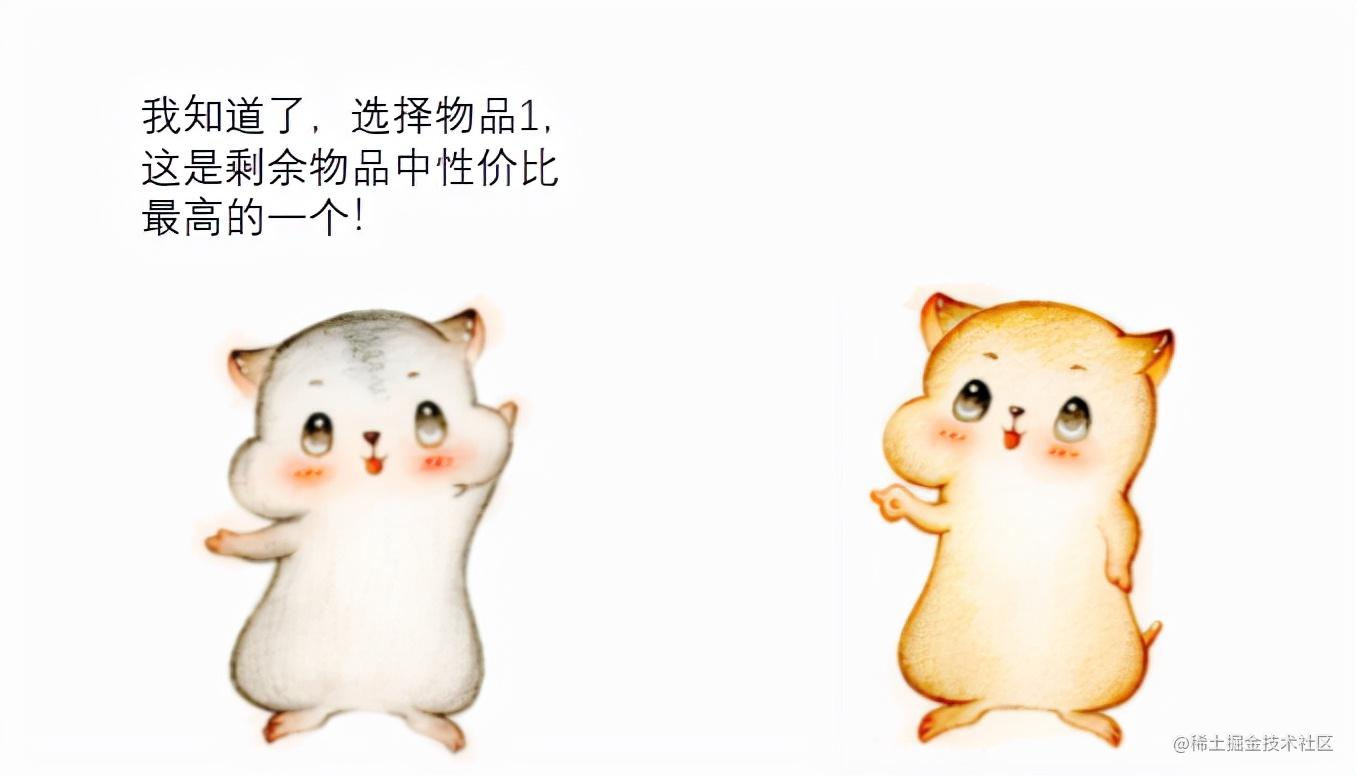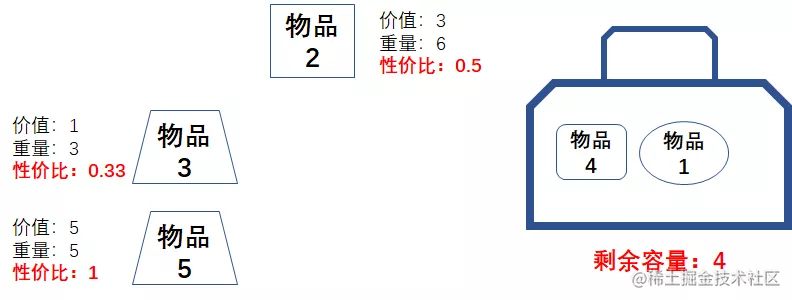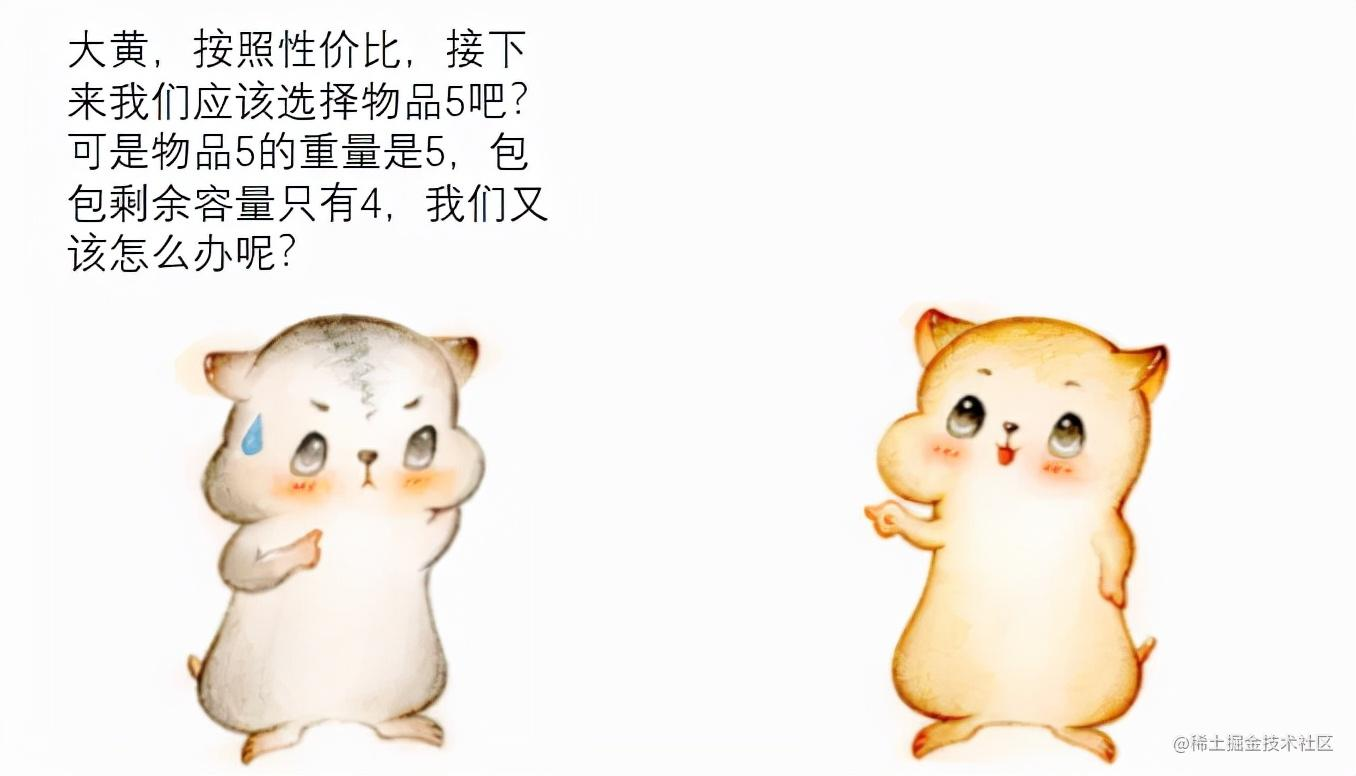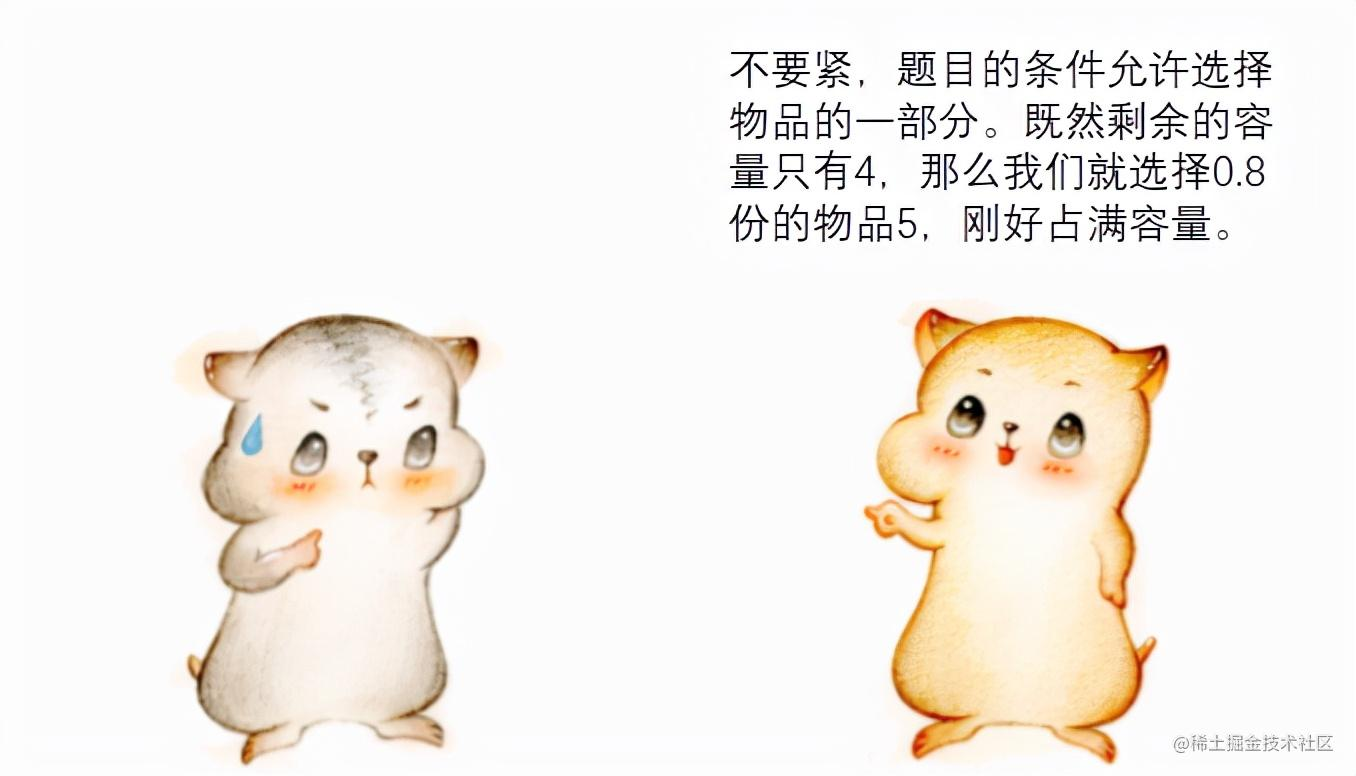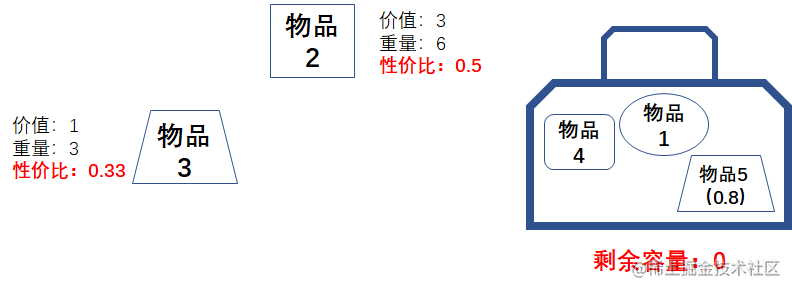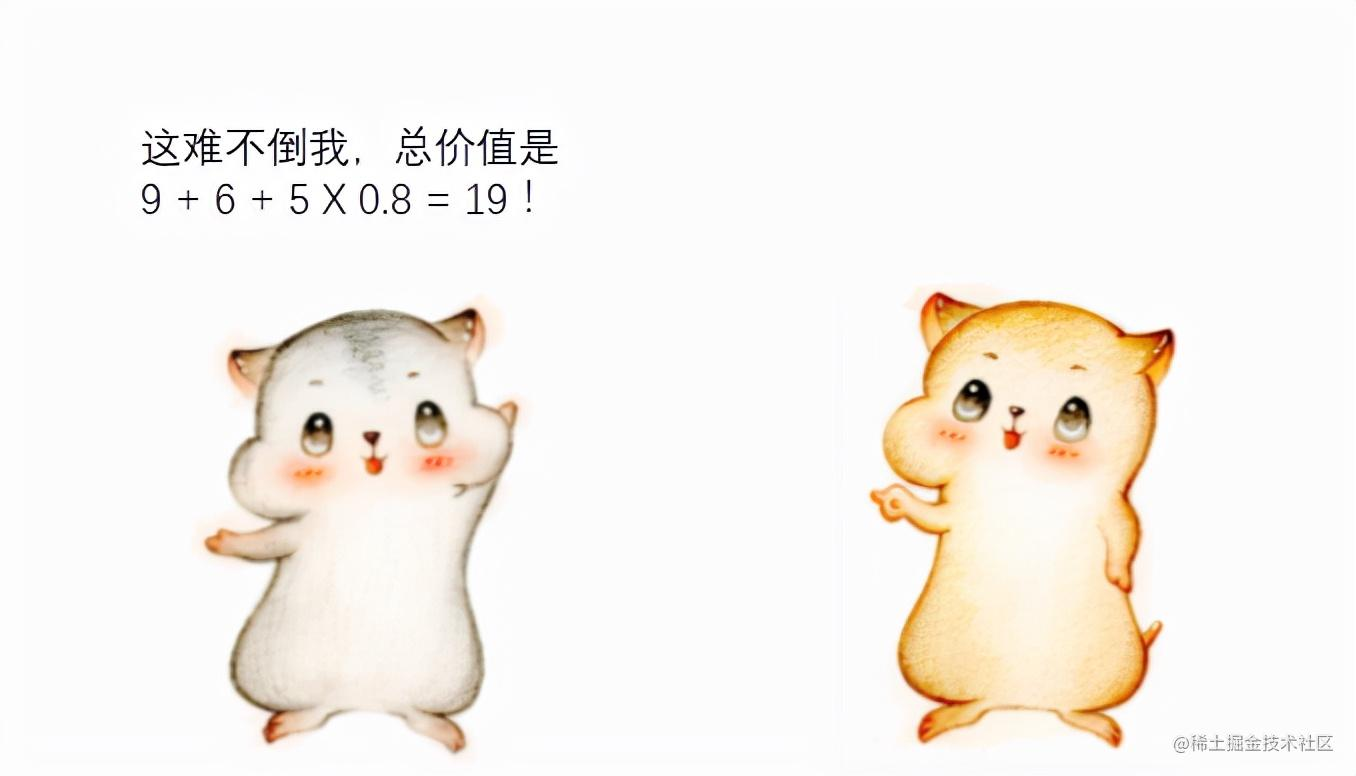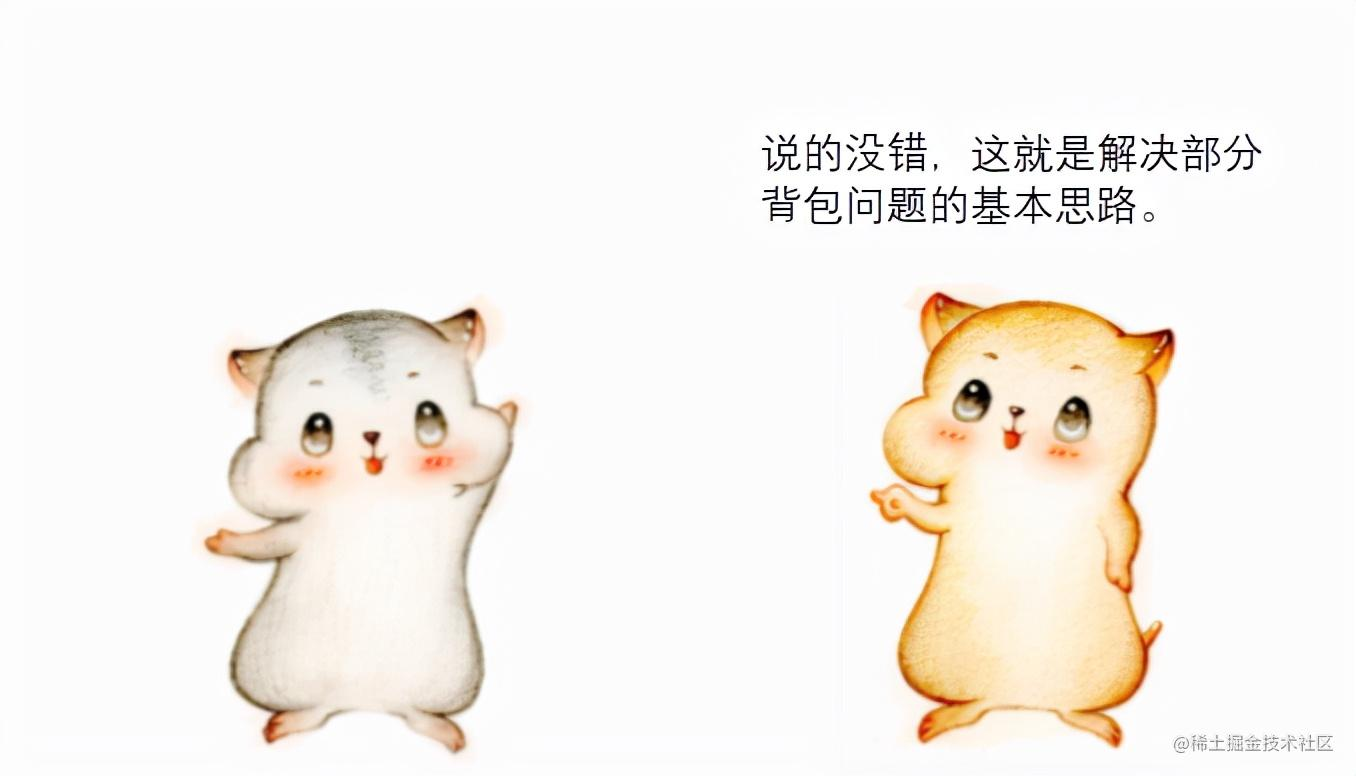public static void main(String[] args) {
int capacity = 10;
int[] weights = {4,6,3,2,4};
int[] values = {9,3,1,6,4};
System.out.println("背包最大价值：" + getHighestValue(capacity, weights, values));
}
public static double getHighestValue(int capacity, int[] weights,int[] values){
//创建物品列表并按照性价比倒序
List itemList = new ArrayList<>();
for(int i=0;i<weights.length;i++){
}
itemList = itemList.stream().sorted(Comparator.comparing(Item::getRatio).reversed()).collect(Collectors.toList());
//背包剩余容量
int restCapacity = capacity;
//当前背包物品的最大价值
double highestValue = 0;
//按照性价比从高到低选择物品
for(Item item : itemList){
if(item.weight <= restCapacity){
highestValue += item.value;
restCapacity -= item.weight;
}else{
//背包装不下完整物品时，选择该件物品的一部分
highestValue += (double)restCapacity / (double)item.weight * item.value;
break;
}
}
return highestValue;
}
static class Item {
private int weight;
private int value;
//物品的性价比
private double ratio;
public Item (int weight, int value){
this.weight = weight;
this.value = value;
this.ratio = (double)value / (double)weight;
}
public double getRatio() {
return ratio;
}
}